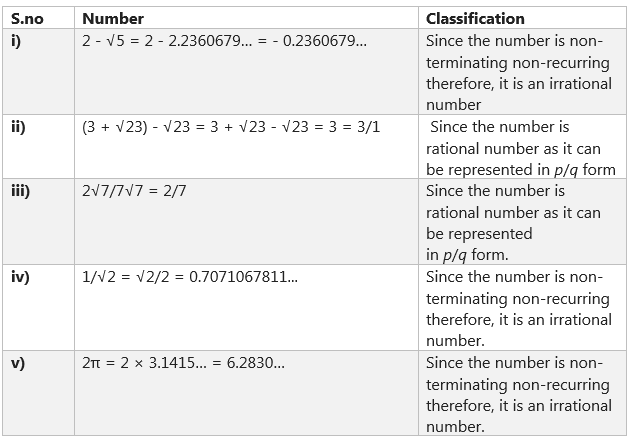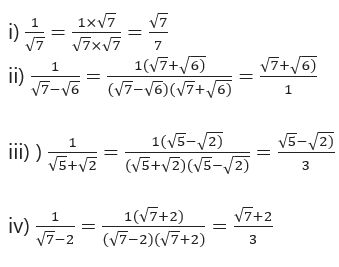# Class 9 maths chapter 1 exercise 1.5 and 1.6 solutions

In this page we have Class 9 maths chapter 1 exercise 1.5 solutions and Class 9 maths chapter 1 exercise 1.6 solutions . Hope you like them and do not forget to like , social share and comment at the end of the page.Free download also available

## Class 9 maths chapter 1 exercise 1.5 solutions

Question 1
Classify the following numbers as rational or irrational:
(i) 2 - √5
(ii) (3 + √23) - √23
(iii) 2√7/7√7
(iv) 1/√2
(v) 2π
SolutionQuestion 2
Simplify each of the following expressions:
(i) (3 + √3) (2 + √2)
(ii) (3 + √3) (3 - √3)
(iii) (√5 + √2)2
(iv) (√5 - √2) (√5 + √2)
Solution
(i) (3 + √3) (2 + √2)
= 3 × 2 + 2 + √3 + 3√2+ √3 ×√2
= 6 + 2√3 +3√2 + √6
(ii) (3 + √3) (3 - √3)
Now as (a + b) (a - b) = a2 - b2
= 32 - (√3)2
= 9 - 3= 6
(iii) (√5 + √2)
Now as (a + b)2 = a2 + b2 + 2ab
= (√5)2 + (√2)2 + 2 ×√5 × √2
= 5 + 2 + 2 × √5× 2
= 7 +2√10

(iv) (√5 - √2) (√5 + √2)
Now as (a + b) (a - b) = a2 - b2
= (√5)2 - (√2)2
= 5 – 2= 3

Question 3
Recall, π is defined as the ratio of the circumference (say c) of a circle to its diameter (say d). That is, π = c/d. This seems to contradict the fact that π is irrational. How will you resolve this contradiction?
Solution
There is no contradiction. When we measure a value with a scale, we only obtain an approximate value. We never obtain an exact value. Therefore, we may not realize that either c or d is irrational. The value of π is almost equal to 22/7 or 3.142857...

Question 4.
Represent √9.3 on the number line.

Question 5
Rationalize the denominators of the following:
(i) 1/√7
(ii) 1/ (√7-√6)
(iii) 1/ (√5+√2)
(iv) 1/ (√7-2)
Solution## Class 9 maths chapter 1 exercise 1.6 solutions

Question 1.
Find:
(i) 641/2
(ii) 321/5
(iii) 1251/3
Solution
(i) 641/2 =(26)1/2  =26 X1/2  = 23 =8
(ii) 321/5  =(25)1/5 = 2
(iii) 1251/3 = (53)1/3 =5

Question 2
Find:
(i) 93/2
(ii) 322/5
(iii) 163/4
(iv) 125-1/3
Solution
(i) 93/2 = (32)3/2 = 27
(ii) 322/5 = (25)2/5 =4
(iii) 163/4 = (24)3/4 =8
(iv) 125-1/3 = 1/1251/3 = 1/(53)1/3 =1/5

Question 3.
Simplify:
(i) 22/3.21/5
(ii) (1/33)7
(iii) 111/2/111/4
(iv) 71/2.81/2
Solution
(i) 22/3.21/5 =2 2/3+ 1/5 = 210+3/15 =213/15
(ii) (1/33)7
=1/33X7 = 1/321 =3 -21
(iii) 111/2/111/4
= 111/2 -1/4  = 111/4
(iv) 71/2.81/2
=(7X8)1/2 = 561/2

## Summary

1. Class 9 maths chapter 1 exercise 1.5 solutions and Class 9 maths chapter 1 exercise 1.6 solutions has been prepared by Expert with utmost care. If you find any mistake.Please do provide feedback on mail. You can download the solutions as PDF in the below Link also
2. This chapter 1 has total 6 Exercise 1.1 ,1.2,1.3 ,1.4 ,1.5 and 1.6. This is the Fifth and Sixth exercise in the chapter.You can explore previous exercise of this chapter by clicking the link below